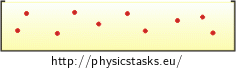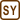## Density of Blood

### Task number: 1757

To determine the density of blood, drops of blood were added to a mixture of xylene with a density of $$0{.}867 \mathrm{\frac{g}{cm^3}}$$and bromobenzene with a density of $$1{.}497 \mathrm{\frac{g}{cm^3}}$$. The ratio of xylene and bromobenzene was being changed until the drops were floating, i. e. they did not rise nor sink. This happened when the mixture contained 72 % of xylene and 28 % of bromobenzene. What was the density of blood?• #### Given values

 $$\rho_{x}={0.867 \mathrm{\frac{g}{cm^3}}}$$ density of xylene $$\rho_{b}=1{.}497 \mathrm{\frac{g}{cm^3}}$$ density of bromobenzene $$V$$ volume of the mixture $$V_{x}=0{.}72 \mathrm{V}$$ volume of xylene $$V_{b}=0{.}28 \mathrm{V}$$ volume of bromobenzene $$\rho_{blood}= ? \mathrm{\frac{g}{cm^3}}$$ density of blood
• #### Analysis

What does it mean that the drops of blood are floating, and what holds for their density in such a situation? After answering these questions, you can determine the density of the mixture.

• #### Hint 1

First remind yourself, how to define the density of a substance.

• #### Hint 2

You know that the blood drops are floating. What applies to the forces acting on the drops in this situation? What can you say about the density of the blood drops?

• #### Hint 3

Choose a volume of the mixture and determine the volume of xylene and bromobenzene.

• #### Solution of Hint 3

For example we choose a volume of $$V=100 \mathrm{cm^3}.$$ Then the volume of xylene is $$V_{x}=0.72{\cdot}100 \mathrm{cm^3}=72 \mathrm{cm^3}$$ and the volume of bromobenzene is $$V_{b}=0{.}28{\cdot}100 \mathrm{cm^3}=28 \mathrm{cm^3}$$.

• #### Hint 4

What is the mass of xylene and bromobenzene (corresponding to the selected volume of the mixture)?

• #### Hint 5

What is the density of the mixture (corresponding to the selected volume of the mixture)?

• #### Overall solution

The definition of density

The density of a substance $$\rho$$ is defined as mass $$m$$ over volume $$V$$: $\rho=\frac{m}{V}.\tag{1}$

The density of blood

Since the blood drops do not sink nor rise in the mixture, the force of gravity $$F_{\mathrm{g}}$$ must be equal to the buoyancy force $$F_{\mathrm{b}}$$: $F_{\mathrm{g}}=F_{\mathrm{b}}.$ Thus: $\rho_{\mathrm{blood}}V_{\mathrm{blood}}g=\rho V_{\mathrm{blood}}g,$ where $$V_{\mathrm{blood}}$$ is the volume of the blood drops, and g is the acceleration of gravity.

By dividing both sides of the equation by $$V_{\mathrm{blood}}g$$ we obtain: $\rho_{\mathrm{blood}}=\rho.$

The density of blood is the same as the density of the mixture.

The volume of xylene and bromobenzene

We choose the volume of the mixture to be $$V=100 \mathrm{cm^3}.$$ Then the volume of xylene is $$V_{\mathrm{x}}=0{.}72{\cdot}100 \mathrm{cm^3}=72 \mathrm{cm^3}$$ and the volume of bromobenzene is $$V_{\mathrm{b}}=0{.}28{\cdot}100 \mathrm{cm^3}=28 \mathrm{cm^3}$$.

The mass of xylene and bromobenzene

From (1) we express a relation for calculating the mass: $m=\rho{\cdot}V.$

Thus, the mass of xylene $$m_{\mathrm{x}}$$ is: $m_{\mathrm{x}}=\rho_{\mathrm{x}}V_{\mathrm{x}}.$ For given values: $m_{\mathrm{x}}=0{.}867{\cdot}72 \mathrm{\frac{g}{cm^3}}\cdot\mathrm{cm^3}=62{.}424 \mathrm{g}.$

The mass of bromobenzene $$m_{\mathrm{b}}$$ is: $m_{\mathrm{b}}=\rho_{\mathrm{b}}V_{\mathrm{b}}.$ For given values: $m_{\mathrm{b}}=1{.}497{\cdot}28 \mathrm{\frac{g}{cm^3}}\cdot\mathrm{cm^3}=41{.}916 \mathrm{g}.$

The density of the mixture

We need to know the total mass of the mixture $$m$$, for which the following applies: $m=m_{x}+m_{b}.$ For given values: $m=62{.}424 \mathrm{g}+41{.}916 \mathrm{g}=104{.}34 \mathrm{g}.$

We have chosen the volume of the mixture to be: $$V=100 \mathrm{cm^3}.$$

By substituting the mass and volume of the mixture into (1),we obtain its density $$\rho$$: $\rho=\frac{104{.}34}{100} \mathrm{\frac{g}{cm^3}}=1{.}0434 \mathrm{\frac{g}{cm^3}} \dot= 1{,}04 \mathrm{\frac{g}{cm^3}}.$

The density of blood is the same as the density of the mixture: $$\rho_{\mathrm{blood}} \dot= 1{.}04 \mathrm{\frac{g}{cm^3}}.$$

The density of blood is the same as the density of the mixture: $$\rho_{\mathrm{blood}} \dot= 1{.}04 \mathrm{\frac{g}{cm^3}}.$$